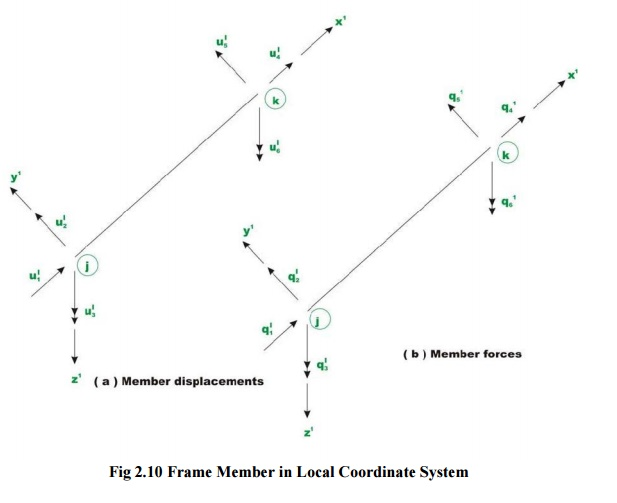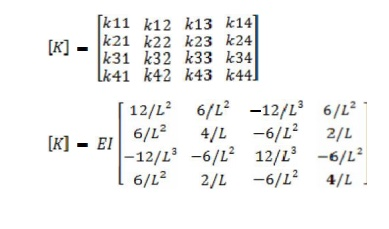Home | | Structural Analysis I | Structural Analysis: Stiffness Matrix Method

# Structural Analysis: Stiffness Matrix Method

Element and global stiffness matrices - Analysis of continuous beams - Co-ordinate transformations - Rotation matrix - Transformations of stiffness matrices, load vectors and displacements vectors - Analysis of pin-jointed plane frames and rigid frames( with redundancy vertical to two)

STIFFNESS MATRIX METHOD

INTRODUCTION

The given indeterminate structure is first made kinematic ally determinate by introducing constraints atthenodes. The required number of constraints is equal to degrees of freedom at the nodes that is kinematic indeterminacy ?k. The kinematic ally determinate structure comprises of fixed ended members, hence, all nodal displacements are zero. These results in stress resultant discontinuities at these nodes under the action of applied loads or in other words the clamped joints are not in equilibrium.

Inorder to restore the equilibrium of stress resultants at the nodes the nodes are imparted suitable unknown displacements. The number of simultaneous equations represen ting joint equilibrium of forces is equal to kinematic indeterminacy ?k. Solution of these equations gives unknown nodal displacements. Using stiffness properties of members the memberend forces are computed and hence the internal forces through out the structure.

Since nodal displacements are unknowns, the method is also called displacement method. Since  equilibrium conditions are applied at the joints the method is also called equilibrium method. Since stiffness properties of members are used the method is also called stiffness method.

In the displacement method of analysis the equilibrium equations are written by expressing the unknown joint displacements in terms of loads by using load-displacement relations. The unknown joint displacements (the degrees of freedom of the structure) are calculated by solving equilibrium equations. The slope -deflection and moment - distribution methods were extensively used before the high speed computing era. After the revolution in computer industry, only direct stiffness method is used.

PROPERTIES OFTHESTIFFNESS MATRIX

The properties of the stiffness matrix are:

It is asymmetric matrix

The sum of elements in any column must be equal to zero.

It is an unstable element therefore the determinant is equal to zero.

ELEMENT AND GLOBAL STIFFNESS MATRICES

Local co ordinates

In the analysis for convenience we fix the element coordinates coincident with the member axis called element (or) local coordinates (coordinates defined along the individual member axis )

Global co ordinates

It is normally necessary to define a coordinate system dealing with the entire structure is called system on global coordinates (Common coordinate system dealing with the entire structure)

Transformation matrix

The connectivity matrix which relates the internal forces Q and the external forces R is known as the force transformation matrix. Writing it in a matrix form,

{Q} =[b]{R}

Where Q=member force matrix/vector, b=force transformation matrix R = external force/load matrix/ vector

ANALYSIS OF CONTINUOUS BEAMSANALYSIS OF PIN JOINTED PLANE FRAMES

An introduction to the stiffness method was given in the previous Page. The basic principles involved in the analysis of beams, trusses were discussed. The problems were solved with hand computation by the direct application of the basic principles. The procedure discussed in the session (page) though      enlighteningarenot suitable for computer programming. It is necessary   to   keesphand computation to a minimum while implementing this procedure on the computer.

In  this session a  formal approach has been  discussed which may  be readily  programmed on  a computer. In this less on the direct stiffness method as applied to planar truss structure is discussed.

Planetrusses are made up of short thin members inter connected a thin gesto form triangulated patterns. Ahinge connection can only transmit forces from one member to another member but not the moment.   For  analysis purpose,  the truss is loaded  at the joints.  Hence,  a truss member is subjected to only axial forces and the forces remain constant along the length of the member. The forces in the member at its two ends must be of the same magnitude but actin the opposite directions for equilibrium as shown in Fig.2.8STIFFNESS MATRIX METHOD

1. What are the basic unknowns in stiffness matrix method?

In the stiffness matrix method nodal displacements are treated as the basic unknowns for the solution of indeterminate structures.

2. Define stiffness coefficient kij.

Stiffness coefficient 'kij' is defined as the force developed at joint 'i' due to unit displacement at joint 'j' while all other joints are fixed.

3. What is the basic aim of the stiffness method?

The aim of the stiffness method is to evaluate the values of generalized coor dinates 'r' knowing the structure stiffness matrix 'k' and nodal loads 'R' through the structure equilibrium equation.

{R} =[K]{r}

4. What is the displacement transformation matrix?

The connectivity matrix which relates the internal displacement 'q' and the external displacement 'r' is known as the displacement transformation matrix 'a'.

{q} =[a]{r}

5. How are the basic equations of stiffness matrix obtained?

The basic equations of stiffness matrix are obtained as:

Equilibrium forces

�Compatibility of displacements

Force displacement relationships

6. What is the equilibrium condition used in the stiffness method?

The external loads and the internal member forces must be in equilibrium at the nodal points.

7. What is meant by generalized coordinates?

For specifying a configuration of a system, a certain minimum no of indepen dent coordinates are necessary. The least no of independent coordinates that are needed to specify the configuration is known as generalized coordinates.

8. What is the compatibility condition used in the flexibility method?

The deformed elements fit together at nodal points.

9. Write about the force displacement relationship.

The relationship of each element must satisfy the stress-strain relationship of the element material.

10.            Write the element stiffness for a truss element.

The element stiffness matrix for a truss element is given by11.            Write the element stiffness matrix for a beam element.

The element stiffness matrix for a beam element is given by12.            Compare flexibility method and stiffness method.

Flexibility matrix method

The redundant forces are treated as basic unknowns.

The number of equations involved is equal to the degree of static indete rminacy of the structure.

The method is the generalization of consistent deformation method. Different procedures are used for determinate and indeterminate structures

Stiffness matrix method

The joint displacements are treated as basic unknowns

The number of displacements involved is equal to the no of degrees of freedom of the structure

The method is the generalization of the slope deflection method.

The same procedure is used for both determinate and indeterminate structures.

13.            Is it possible to develop the flexibility matrix for an unstable structure?

In order to develop the flexibility matrix for a structure, it has to be stable and determinate.

14.            What is the relation between flexibility and stiffness matrix?

The element stiffness matrix 'k' is the inverse of the element flexibility matrix 'f' and is given by f=1/k or k =1/f.

15.            What are the type of structtures that can be solved using stiffness matrix method?

Structures such as simply supported, fixed beams and portal frames can be solved using stiffness matrix method.

16.            Give the formula for the size of the Global stiffness matrix.

The size of the global stiffness matrix (GSM) =No: of nodes x Degrees of free dom per node.

17.            List the properties of the stiffness matrix

The properties of the stiffness matrix are:

It is a symmetric matrix

The sum of elements in any column must be equal to zero.

It is an unstable element there fore the determinant is equal to zero.

18Why is the stiffness matrix method also called equilibrium method or displacement method?

Stiffness method is based on the superposition of displacements and hence is also known as the dispalcement method. And since it leads to the equilibrium equations the method is also known as equilibrium method.

19 If the flexibility matrix is given as20 Write the n stiffness matrix for a 2D beam element. The stiffness matrix for a 2 D beam element is given byStudy Material, Lecturing Notes, Assignment, Reference, Wiki description explanation, brief detail
Civil : Structural Analysis : Stiffness Matrix Method : Structural Analysis: Stiffness Matrix Method |